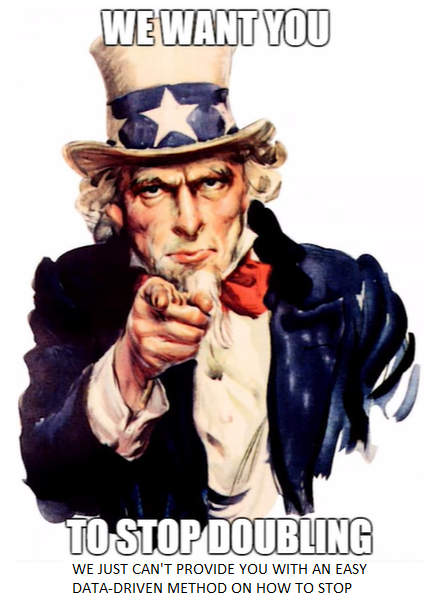# Doing A Double-Take At Predicting Doubles

Sean has become insufferable since my last article. He send me things like this now: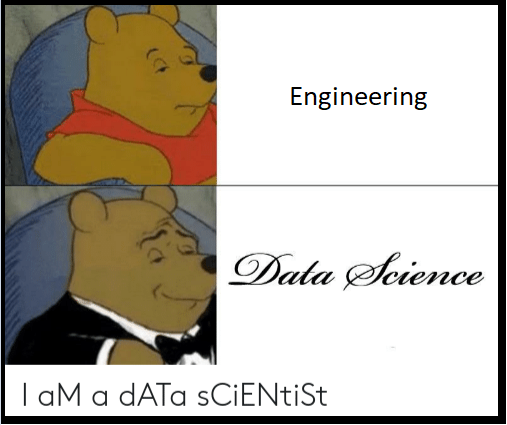Editors Note: Slander. Even if you don’t believe I’m nice to all my contributors there is no way in a million years I would make a meme that had that annoying alternating caps text. (I will, however, admit that all engineers feel that we can do everyone else’s profession better than they can.)

I need to redeem myself, so I thought of another way to predict doubles. What if instead of trying to predict how many doubles will occur in a match or tournament, we look at if we can predict if a specific exchange will result in a double? This sounds way more cool, and potentially a more coachable way of looking at doubles.

The same HEMA Scorecard data that was used for the prior article was also used for this attempt as well. As such, it would make sense for you to review that article for some of the data nuances.

## The Data

Because the same data is being used as last time, we have the exact same counts as before: 12,772 matches from 277 tournaments at 80 events. However, this time we are looking one layer deeper: the exchange. Scorecard has provided information for a whopping 79,976 exchanges.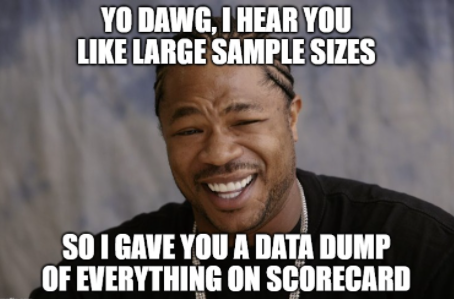We are using variables that are very similar to the models from the previous article, with the addition of some new ones that we can calculate because we are looking at this at the exchange-level. Here’s an overview of the variables involved:

You might notice that there is a “max” and “min” version for several of these variables. The reason behind this is that we have a “Fighter 1” and “Fighter 2” for each match; because being assigned to 1 or 2 is arbitrary, you should not assign values to “Fighter 1” and “Fighter 2”. Therefore, you have to figure out ways to make the variables independent of being 1 or 2. Taking the minimum and maximum of the variable you are looking at, such as current points or prior matches, will allow for this sort of independence.

## Modeling

Because what we want to predict is a double or no double for the next exchange, what we are dealing with is a binary variable. If you recall from this article, logistic regression, decision trees, and ensemble methods are good models to use for binary variables. I tried all of them, and none of them produced good results. However…

There’s something called a Support Vector Machine, shortened to SVM, which did perform decently. To explain it simply, an SVM tries to find a boundary between different classes; in this case, the classes would be double and not-a-double. For an easy example with just two predictor variables, an SVM can be visualized like this: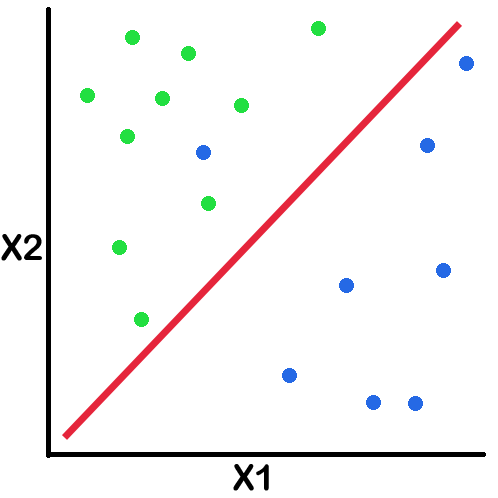As you can see, the greens and blues are divided pretty well by the red line. This is essentially what an SVM does. However, the SVM that I created uses way more than 2 predictor variables, and also isn’t built on a linear algorithm, so it can’t be visualized like the above.

## Model Performance

To understand the model’s performance, we need to talk about something called a confusion matrix. The confusion matrix represents true positives, false positives, true negatives, and false negatives. To explain these, we’ll consider a situation where the line judges are calling doubles and the following scenarios:

A confusion matrix will look like this, but with numbers:

Or, if you like pictures better, we can look at this one: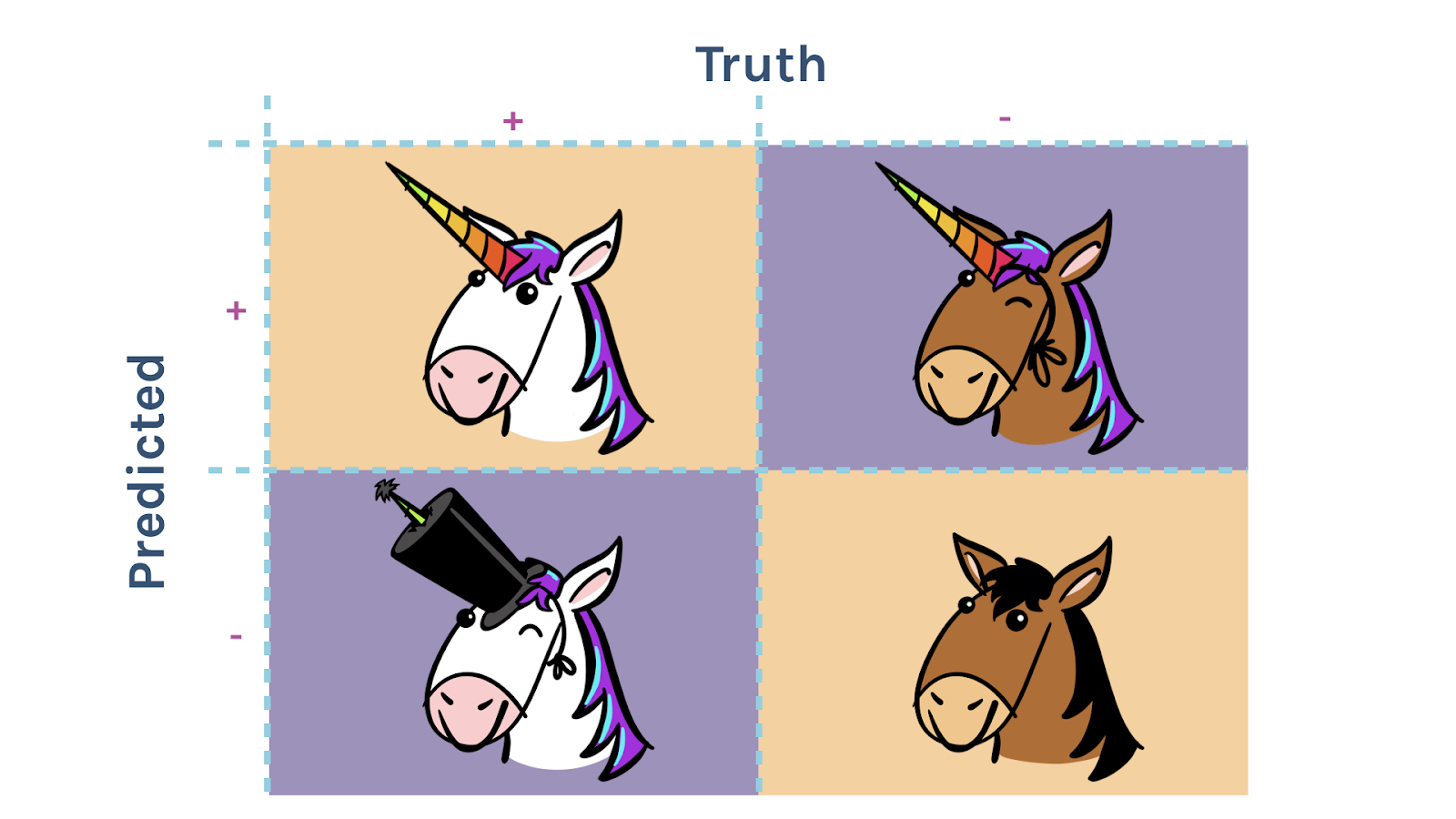The confusion matrix for the testing data in our model looks like this:

But what does this tell us? One of the first metrics we can derive from the confusion matrix is accuracy. Accuracy will tell us how often the model predicts something correctly. For doubles, this means how often was a double predicted as a double and a non-double predicted as a non-double. The formula for this is (True Positive + True Negative)/(Total). Our model has an accuracy of 75.3%. YAY! This is a pretty decent accuracy!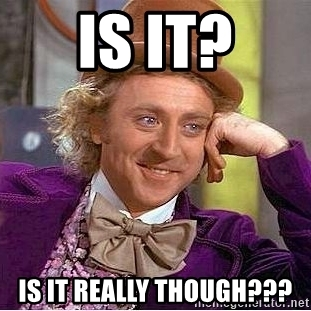In models where the thing we want to predict is about half of the data, this would be good accuracy. However, with our exchanges data, doubles only make up about 15% of the data, leaving non-doubles at 85%. Let’s just pretend that we predicted everything as a non-double. This would mean that the model’s accuracy would be 85%. Hmmm.

Fortunately we have a couple other metrics as well, called precision and recall.

• Precision tells us how often the things we predict as doubles are actually doubles, with the formula being (True Positive)/(True Positive + False Positive).
• Recall tells us how many of the doubles we actually predicted correctly, with the formula being (True Positive)/(True Positive + False Negative).

These are very important metrics because they tell you how good you are at predicting the thing you actually want to predict. This SVM gives us a 32.7% precision and 62.8% recall. These look a bit low…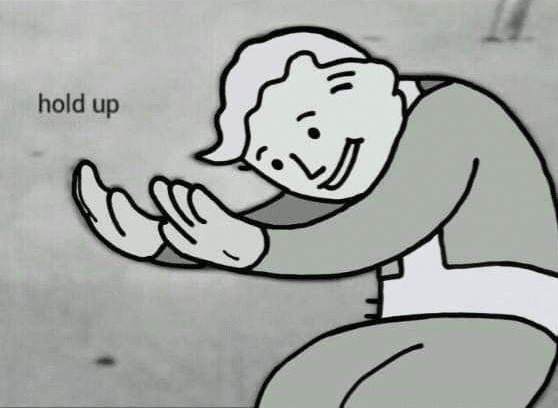Actually, not quite. Let’s take into consideration three different scenarios.

1. A random person comes in off the street because they saw people with swords hanging out in the reception area of the hotel and thought it looked cool. This person wants to predict doubles. Not knowing any better, he might think there’s a 50/50 chance of getting a double because there are only two choices: double or no double. This person would have an accuracy of 50%, a precision of 15%, and a recall of 50%.
2. A beginner HEMA practitioner decided to attend an event to support her team despite not fighting in any tournament herself. Because she has sparred before, she knows that doubles don’t occur at a high rate, and may even know they are a 15/85 split. This person would have an accuracy of 75%, a precision of 15%, and a recall of 15%.
3. A seasoned HEMA practitioner has decided to make a bet with someone else that he can predict doubles versus non-doubles. He wins \$1 every time his prediction is accurate and loses \$1 every time it is inaccurate. Knowing that non-doubles occur far more than doubles, he decides the safest course is to just predict everything as a non-double. He would have 85% accuracy, 0% precision, and 0% recall.

As you can see from the above examples, my SVM has better accuracy in two cases, with better precision and recall than any of these types of guess work.

We also have a measurement that tells us how well the model performs at prediction. This measurement is called an ROC Curve and can be used to compute a metric called the Area Under the Curve, or AUC. The closer the AUC is to 1, the better the model is at predicting the outcome variable; any model with above a 0.7 AUC is considered an acceptable model. In the case of this SVM, the AUC is 0.702– success! We can visualize the ROC Curve and AUC in a graph like this: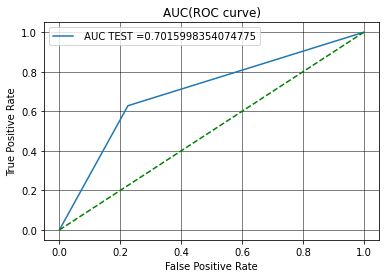Because mathematicians aren’t very creative with their naming, it might be easy to see how AUC is calculated from this image. Basically, it is the area under the blue line on the graph. The dotted green line represents how well random chance would do at predicting a double; the AUC of the green line is 0.5.

## Can This Teach Me How to Recognize a Doubling Situation?You thought you’d be able to take this model and use it to help you gain some competitive advantage, didn’t you? Sorry to burst your bubble, but you can’t.

As I mentioned earlier, this SVM is not based on a linear algorithm. I literally broke the algorithm when trying to find a linear SVM because the data just wasn’t suited to that type of model. Instead, it uses something called a Radial Basis Function, or RBF, to find the boundaries. I would explain it, but believe me, you don’t want me to explain it. Because the SVM uses this kind of algorithm, the effect each input variable has on predicting a double is locked away in a black box.

“A black box that gets it right a decent chunk of the time, but has a chance of being wrong on occasion for unknowable reasons, and is completely incomprehensible to us. I think you just described a tournament judge.”

-Sean Franklin*

## Final Thoughts

• Hours Spent on this Article, Converted to Federal Minimum Wage: \$326.50
• Sunk Cost on HEMA Practices Missed Due to Data Obsession: \$14.25
• Dollars Spent on Caffeine to Stay Up Until 3:00 AM Writing Code: \$16.00
• Proving Sean Wrong: Priceless

Predicting doubles can be done to a certain extent! Data Science wins! However, this is not a very practical model because it doesn’t provide insight into which factors contribute to doubles. The best you could hope for is someone to create a program where it crunches the numbers from the current exchange for you. Wouldn’t it be great to have the tournament table staff yell out “Double Predicted!” to give you a warning? Sounds like it’s time for a new Scorecard feature!

One last message for all you competitors out there: